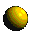# Validation and Verification of Neural Network Systems

Publications

All the publications below are available in compressed postscript.Using Bayesian Neural Networks to Classify segmented Images
5th IEE International conference on Artificial Neural Networks (Cambridge, 7-9 July 1997)

 Abstract. In this paper we present results that compare the performance of neural networks trained with two Bayesian methods, (i) the Evidence Framework of MacKay (1992) and (ii) a Markov Chain Monte Carlo method due to Neal (1996) on a task of classifying segmented outdoor images. We also investigate the use of the Automatic Relevance Determination method for input feature selection.Upper and lower bounds on the learning curve for Gaussian processes
Submitted to Machine Learning

 Abstract. In this paper we introduce and illustrate non-trivial upper and lower bounds on the learning curves for one-dimensional Gaussian Processes. The analysis is carried out emphasising the effects induced on the bounds by the smoothness of the random process described by the Modified Bessel and the Squared Exponential covariance functions. We present an explanation of the early, linearly-decreasing behaviour of the learning curves and the bounds as well as a study of the asymptotic behaviour of the curves. The effects of the noise level and the lengthscale on the tightness of the bounds are also discussed.Discovering hidden features with Gaussian processes regression
To appear in: Advances in Neural Information Processing Systems 11, eds. M. J. Kearns, S. A. Solla and D. A. Cohn. MIT Press, 1999

 Abstract. In Gaussian process regression the covariance between the outputs at input locations x and x' is usually assumed to depend on the distance (x-x')^T W (x-x') where W is a positive definite matrix. W is often taken to be diagonal, but if we allow W to be a general positive definite matrix which can be tuned on the basis of training data, then an eigen-analysis of W shows that we are effectively creating hidden features, where the dimensionality of the hidden-feature space is determined by the data. We demonstrate the superiority of predictions using the general matrix over those based on a diagonal matrix on two test problems.

## Contact names

Francesco Vivarelli
Dr. Christopher K. I. Williams
Dr. W. Andrew Wright